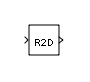• Library:

•## Description

The Radians to Degrees block computes the output angle in degrees from the input angle in radians.

degrees = (180/ n ) × radians

degrees is the angle in degrees and radians is the angle in radians.

## Ports

### Input

expand all

Input signal specifying the angle in radians.

Data Types: `single` | `double` | `int8` | `int16` | `int32` | `uint8` | `uint16` | `uint32` | `fixed point`

### Output

expand all

Output signal specifying the input angle in degrees.

Data Types: `single` | `double` | `int8` | `int16` | `int32` | `uint8` | `uint16` | `uint32` | `fixed point`

## Version History

Introduced in R2008b